# 深度学习中的微分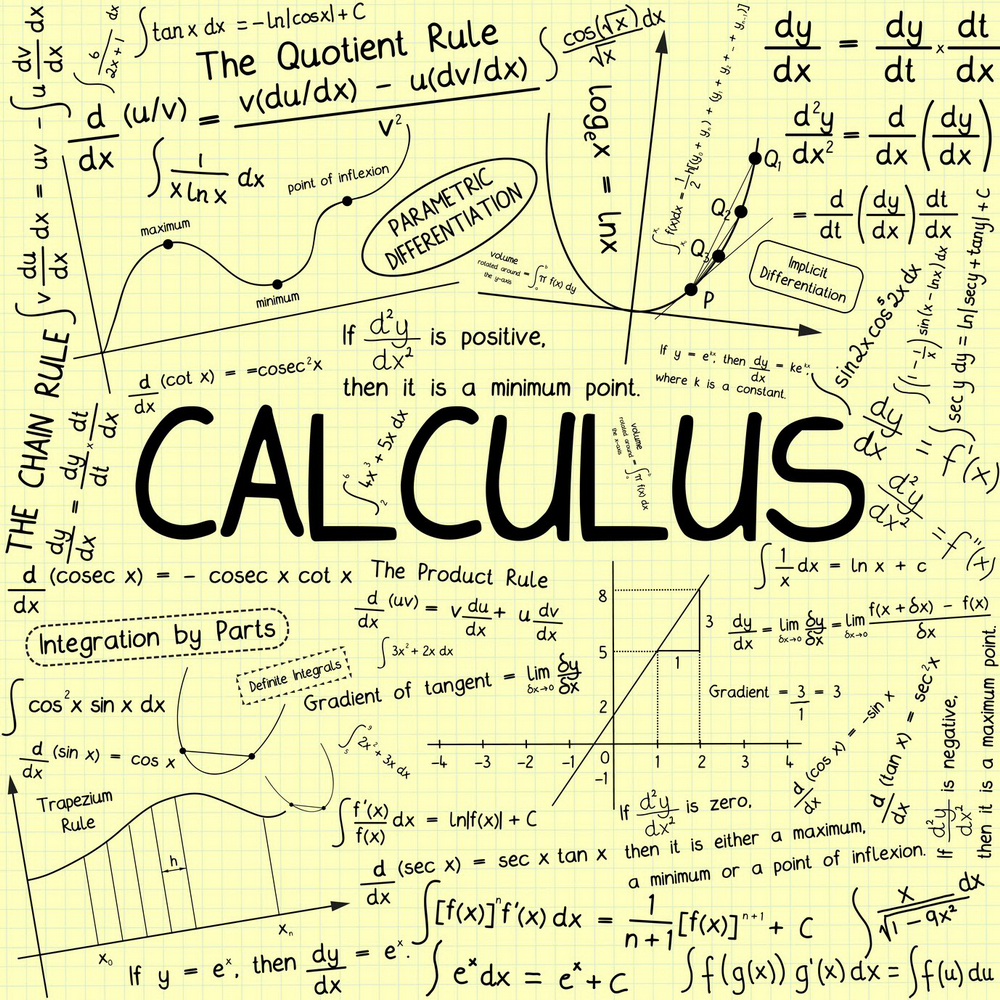## Manual Differentiation

Manual Differentiation 正如字面意思所写，就是手动对函数求导。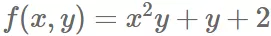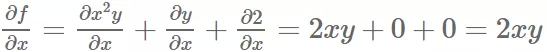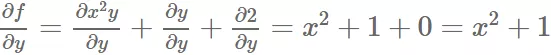## Symbolic Differentiation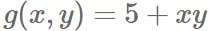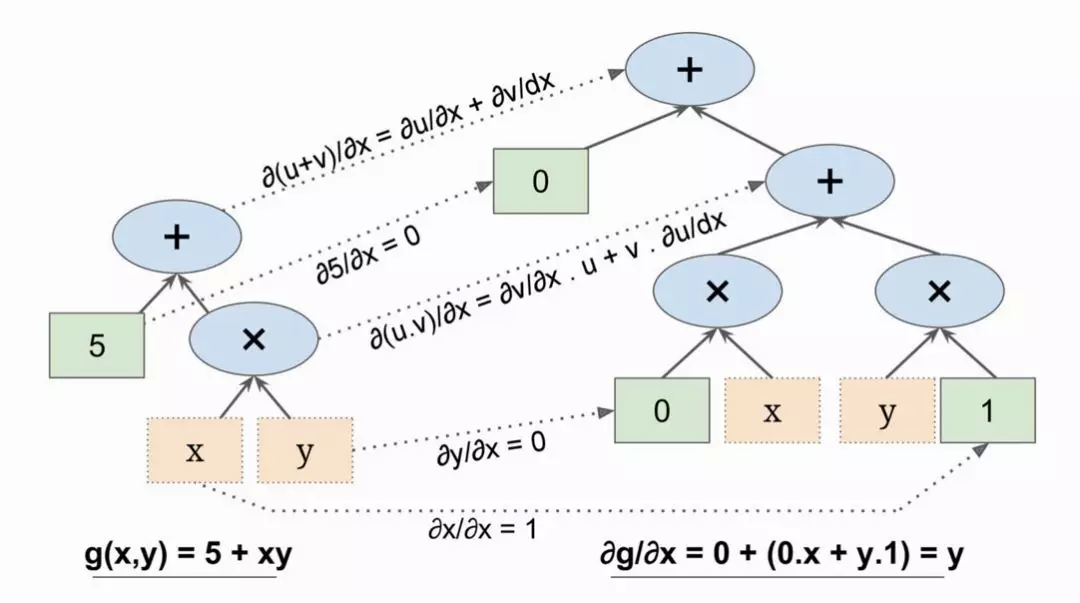def my_func(a, b):    z = 0    for i in range(1000):        z = a * np.cos(z + i) + z * n    return z

## Numeric Differentiation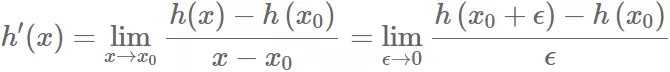def f(x, y):    return x**2*y + y + 2    def derivative(f, x, y, x_eps, y_eps):    return (f(x + x_eps, y + y_eps) - f(x, y)) / (x_eps + y_eps) df_dx = derivative(f, 3, 4, 0.00001, 0) # 24.000039999805264df_dy = derivative(f, 3, 4, 0, 0.00001) # 10.000000000331966

## Automatic Differentiation

Autodiff 算是在之前提到的这些方案基础之上得到的一个兼具性能和数值稳定的方案。

1. Forward-Mode Autodiff

• Forward-Mode 是数值微分和符号微分的结合
• 引入二元数ε， ϵ**2 = 0 ( ϵ ≠ 0)
•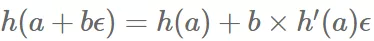• 所以 f(x,y) 在 (3,4) 点对 x 的导数为ϵ前面的系数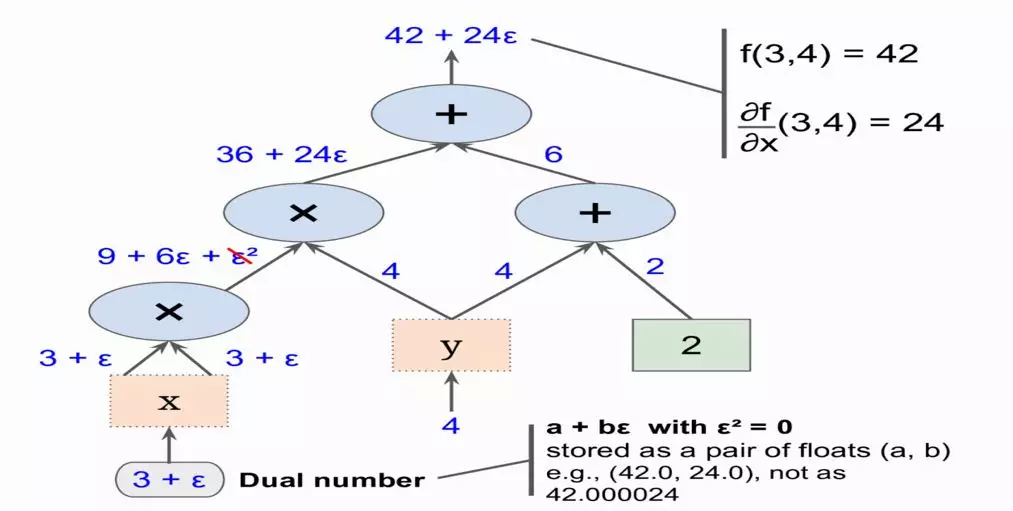forward-mode autodiff 比数值微分更准确，但是也有同样的问题，就是有 n 个参数要走 n 次图

2. Reverse-Mode Autodiff

• 第一遍，先从输入到输出，进行前向计算
• 第二遍，从输出到输入，进行反向计算求偏导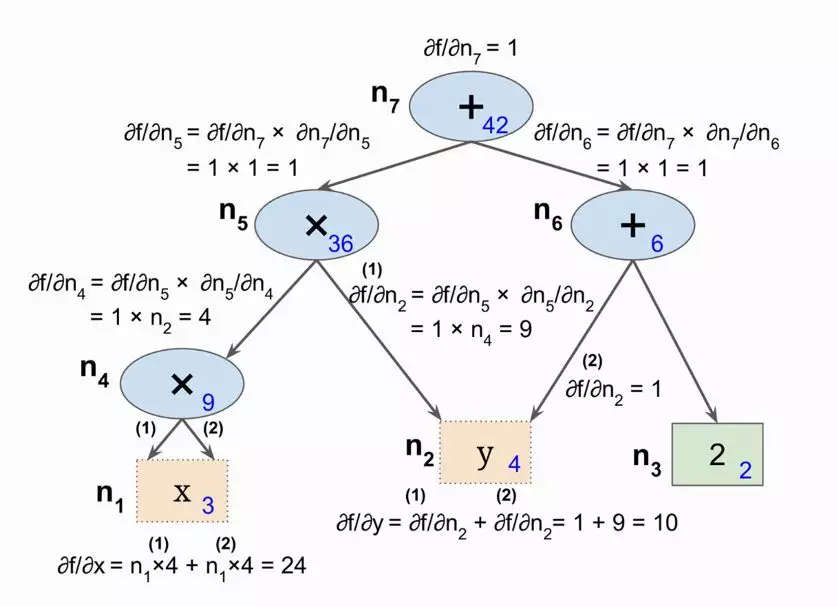• Reverse-Mode Autodiff 在有大量输入，少量输出时是非常有力的方案

• 一次正向，一次反向可以计算所有输入的偏导

https://github.com/mattjj/autodidact

3. 相关工程实现

Autograd 将 numpy 包了一层，提供与 numpy 相同的基础 ops，但自己内部创建了计算图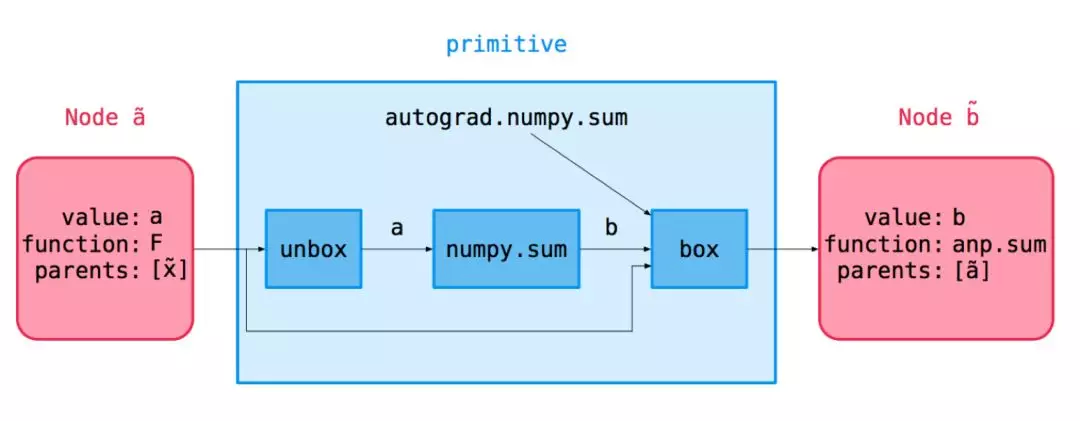import autograd.numpy as npfrom autograd import grad  def logistic(z):    # 等价形式    # np.reciprocal(np.add(1, np.exp(np.negative(z))))    return 1. / (1. + np.exp(-z)) print(logistic(1.5))                              # 0.8175744761936437print(grad(logistic)(1.5))                    # 0.14914645207033284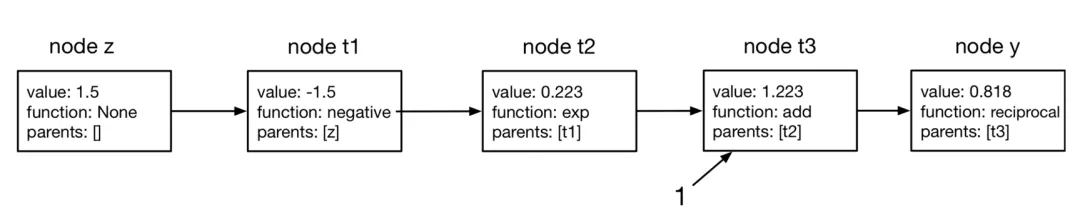① Vector-Jacobian Products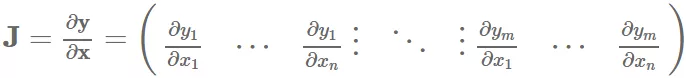vector-Jacobian product (VJP)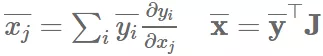primitive_vjps = defaultdict(dict)def defvjp(fun, *vjps, **kwargs):    argnums = kwargs.get('argnums', count())    for argnum, vjp in zip(argnums, vjps):        primitive_vjps[fun][argnum] = vjp  defvjp(anp.negative, lambda g, ans, x: -g)defvjp(anp.exp,    lambda g, ans, x: ans * g)defvjp(anp.log,    lambda g, ans, x: g / x)defvjp(anp.tanh,   lambda g, ans, x: g / anp.cosh(x) **2)defvjp(anp.sinh,   lambda g, ans, x: g * anp.cosh(x))defvjp(anp.cosh,   lambda g, ans, x: g * anp.sinh(x))

tracing the forward pass to build the computation graph 上边的代码是截取了 autodidact 的部分代码，像 negative 基本操作，求出倒数就是原来基础上取负。整个过程可以划分为三块。

• vector-Jacobian products for primitive ops
• backwards pass

## Reference

1. Géron, Aurélien. “Hands-On Machine Learning with Scikit-Learn and TensorFlow”

https://www.cs.toronto.edu/~rgrosse/courses/csc321_2018/slides/lec10.pdf